# Scheduling in Greedy Algorithms

In this article, we will discuss various scheduling algorithms for Greedy Algorithms. Many scheduling problems can be solved using greedy algorithms.

Problem statement: Given N events with their starting and ending times, find a schedule that includes as many events as possible. It is not possible to select an event partially. Consider the below events: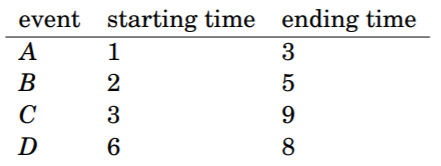• In this case, the maximum number of events is two. As selected events B and D are as follows: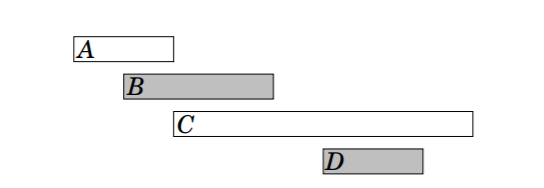• It is possible to invent several greedy algorithms for the problem.

Algorithms that work with every case:

Algorithm 1

• The first idea is to select as short events as possible. In the example, this algorithm selects the following events: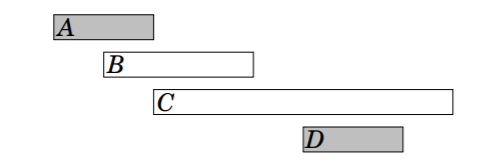• However, selecting short events is not always a correct strategy. For example, the algorithm fails in the below case:• If short event is selected, it can only select one event. However, it would be possible to select both long events.

Algorithm 2:

• Another idea is to always select the next possible event that begins as early as possible. This algorithm selects the following events: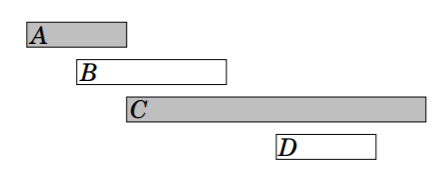• However, given a counter example for this algorithm. In this case, the algorithm only selects one event:• If the first event is selected, it is not possible to select any other events. However, it would be possible to select the other two events.

Algorithm 3:

• The third idea is to always select the next possible event that ends as early as possible. This algorithm selects the following events: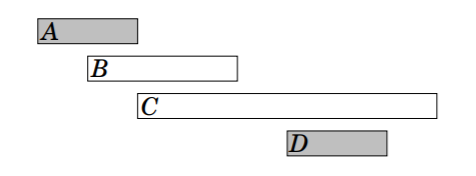• It turns out that this algorithm always produces an optimal solution.
• The reason for this is that it is always an optimal choice to first select an event that ends as early as possible.
• After this, it is an optimal choice to select the next event using the same strategy, etc., until any other event can’t be selected.
• One way the algorithm works is to consider what happens if first select an event that ends later than the event that ends as early as possible.
• Now, with having at most an equal number of choices how the next event can be selected.
• Hence, selecting an event that ends later can never yield a better solution, and the greedy algorithm is correct.
Feeling lost in the world of random DSA topics, wasting time without progress? It's time for a change! Join our DSA course, where we'll guide you on an exciting journey to master DSA efficiently and on schedule.
Ready to dive in? Explore our Free Demo Content and join our DSA course, trusted by over 100,000 geeks!